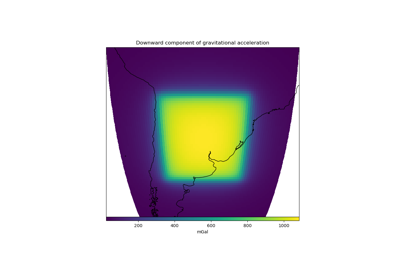# harmonica.tesseroid_gravity¶

`harmonica.``tesseroid_gravity`(coordinates, tesseroids, density, field, parallel=True, radial_adaptive_discretization=False, dtype=<class 'numpy.float64'>, disable_checks=False)[source]

Compute gravitational field of tesseroids on computation points.

Warning

The `g_z` field returns the downward component of the gravitational acceleration on the local North oriented coordinate system. It is equivalent to the opposite of the radial component, therefore it’s positive if the acceleration vector points inside the spheroid.

Parameters
• coordinates (list or 1d-array) – List or array containing `longitude`, `latitude` and `radius` of the computation points defined on a spherical geocentric coordinate system. Both `longitude` and `latitude` should be in degrees and `radius` in meters.

• tesseroids (list or 1d-array) – List or array containing the coordinates of the tesseroid: `w`, `e`, `s`, `n`, `bottom`, `top` under a geocentric spherical coordinate system. The longitudinal and latitudinal boundaries should be in degrees, while the radial ones must be in meters.

• density (list or array) – List or array containing the density of each tesseroid in kg/m^3.

• field (str) –

Gravitational field that wants to be computed. The available fields are:

• Gravitational potential: `potential`

• Downward acceleration: `g_z`

• parallel (bool (optional)) – If True the computations will run in parallel using Numba built-in parallelization. If False, the forward model will run on a single core. Might be useful to disable parallelization if the forward model is run by an already parallelized workflow. Default to True.

• radial_adaptive_discretization (bool (optional)) – If `False`, the adaptive discretization algorithm will split the tesseroid only on the horizontal direction. If `True`, it will perform a three dimensional adaptive discretization, splitting the tesseroids on every direction. Default `False`.

• dtype (data-type (optional)) – Data type assigned to the resulting gravitational field. Default to `np.float64`.

• disable_checks (bool (optional)) – Flag that controls whether to perform a sanity check on the model. Should be set to `True` only when it is certain that the input model is valid and it does not need to be checked. Default to `False`.

Returns

result (array) – Gravitational field generated by the tesseroids on the computation points.

Examples

```>>> # Get WGS84 ellipsoid from the Boule package
>>> import boule
>>> ellipsoid = boule.WGS84
>>> # Define tesseroid of 1km of thickness with top surface on the mean
>>> thickness = 1000
>>> bottom = top - thickness
>>> w, e, s, n = -1.0, 1.0, -1.0, 1.0
>>> tesseroid = [w, e, s, n, bottom, top]
>>> # Set a density of 2670 kg/m^3
>>> density = 2670.0
>>> # Define computation point located on the top surface of the tesseroid
>>> coordinates = [0, 0, ellipsoid.mean_radius]
## Examples using `harmonica.tesseroid_gravity`¶